# Divide up to 4 digits by 1 digit

## Learning focus

Learn how to divide up to 4-digit numbers by 1-digit numbers.

This includes:

• a learning summary
• one video
• one slideshow
• two activities

# Quiz

To get started, let's see how well you know this topic already. Take the quiz below to find out.

# Learn

Find out about dividing a 4-digit number by a 1-digit number with this video.

Learn more about how to break down a calculation when dividing a 4-digit number by a 1-digit number: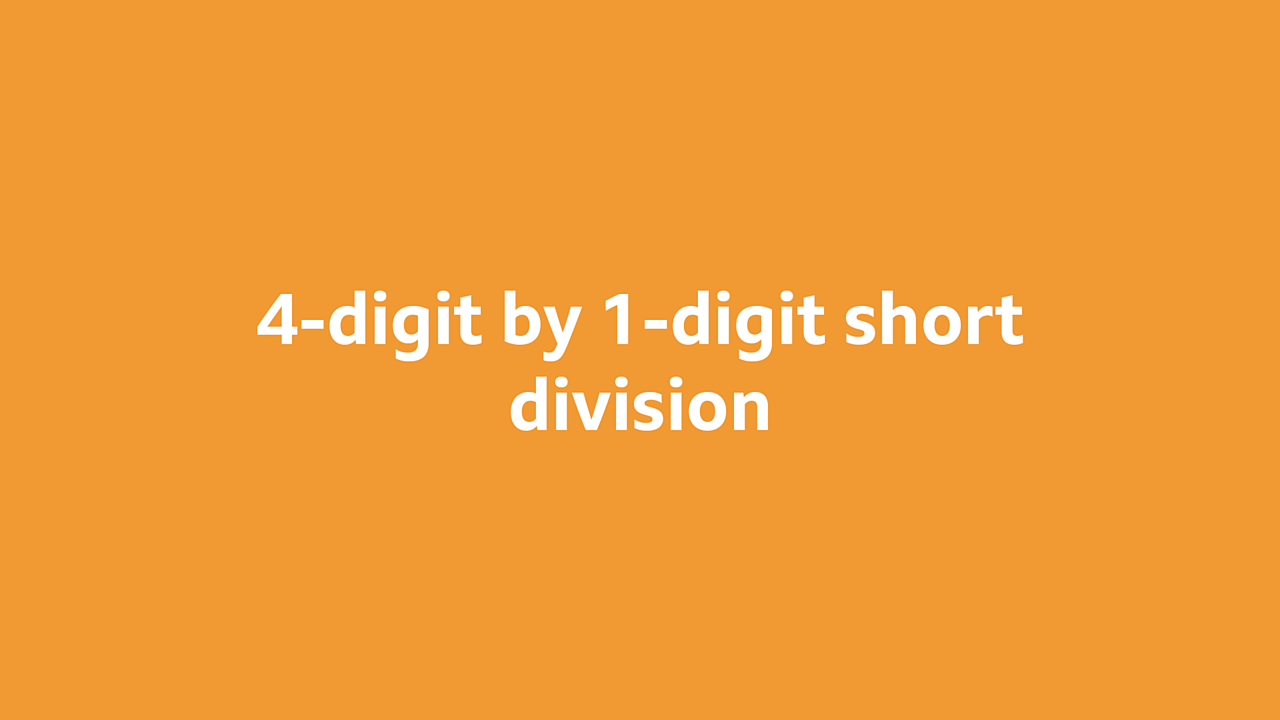4-digit by 1-digit short division1 of 8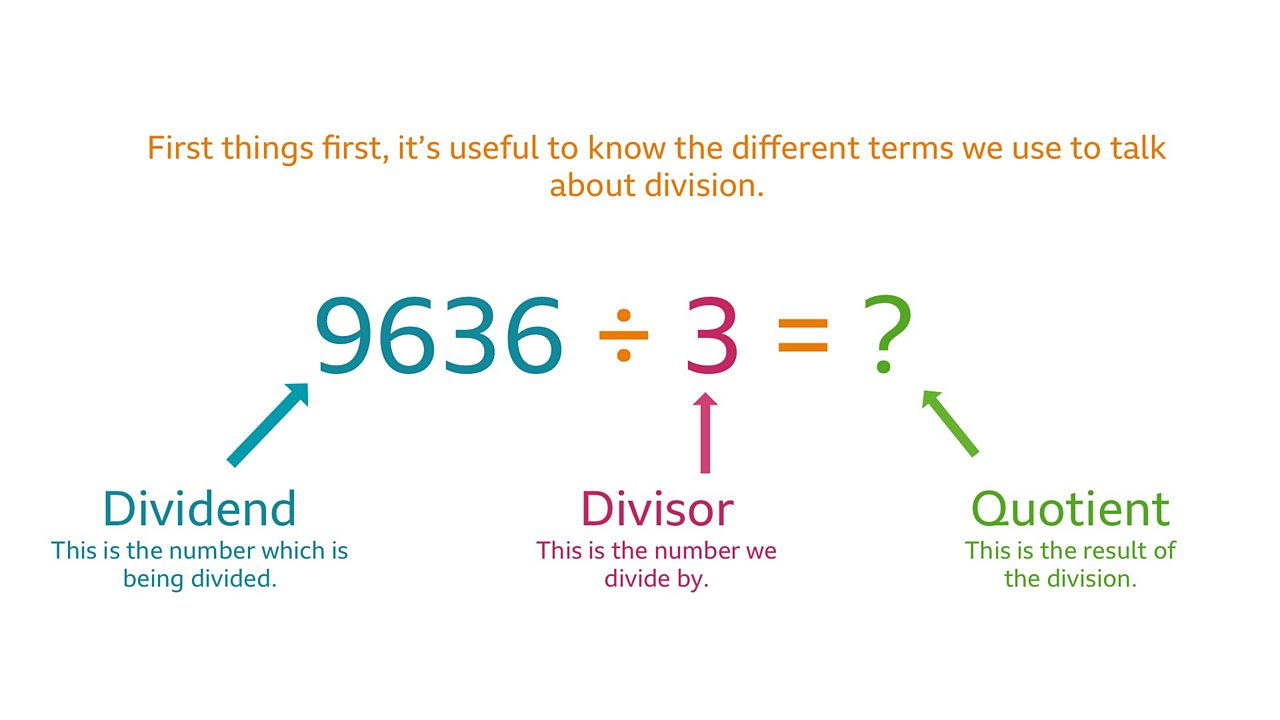In division, we talk about the dividend (the number being divided) the divisor (the number we divide by) and the quotient (the result of the division.)2 of 8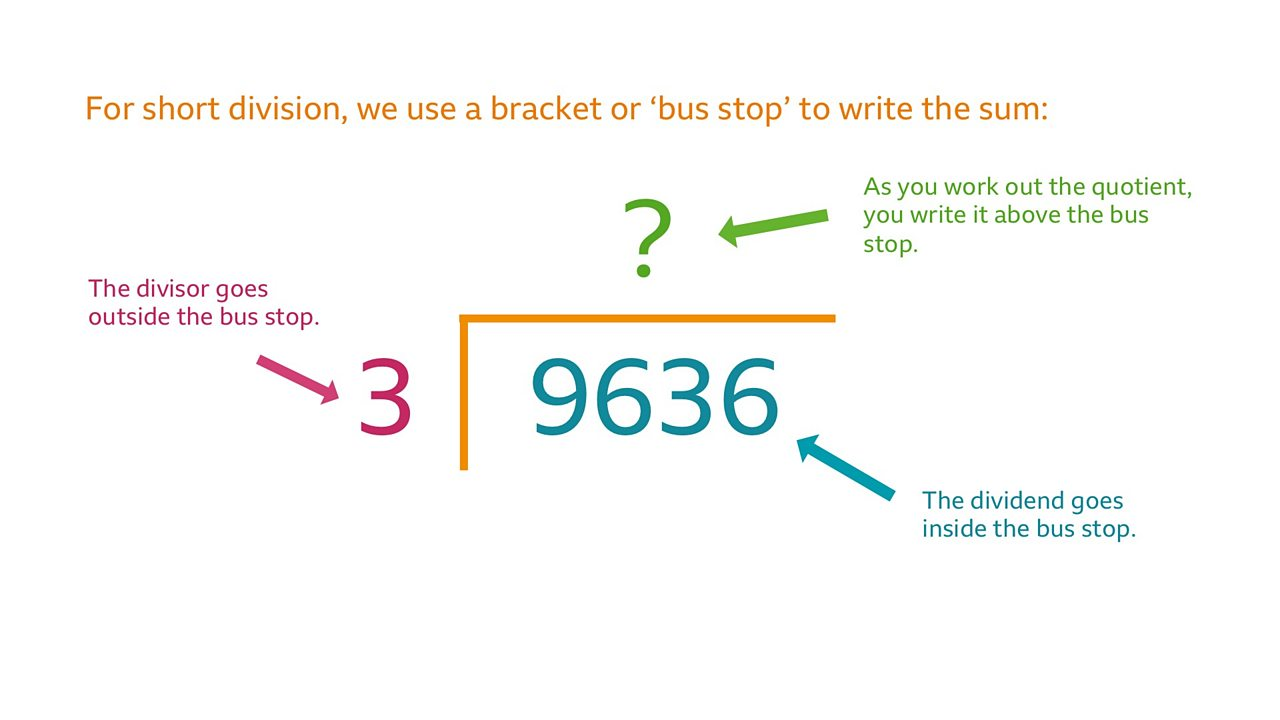For short division, we use a bracket or 'bus stop' to write the sum. The divisor goes outside the bus stop, the dividend goes inside, the quotient above.3 of 8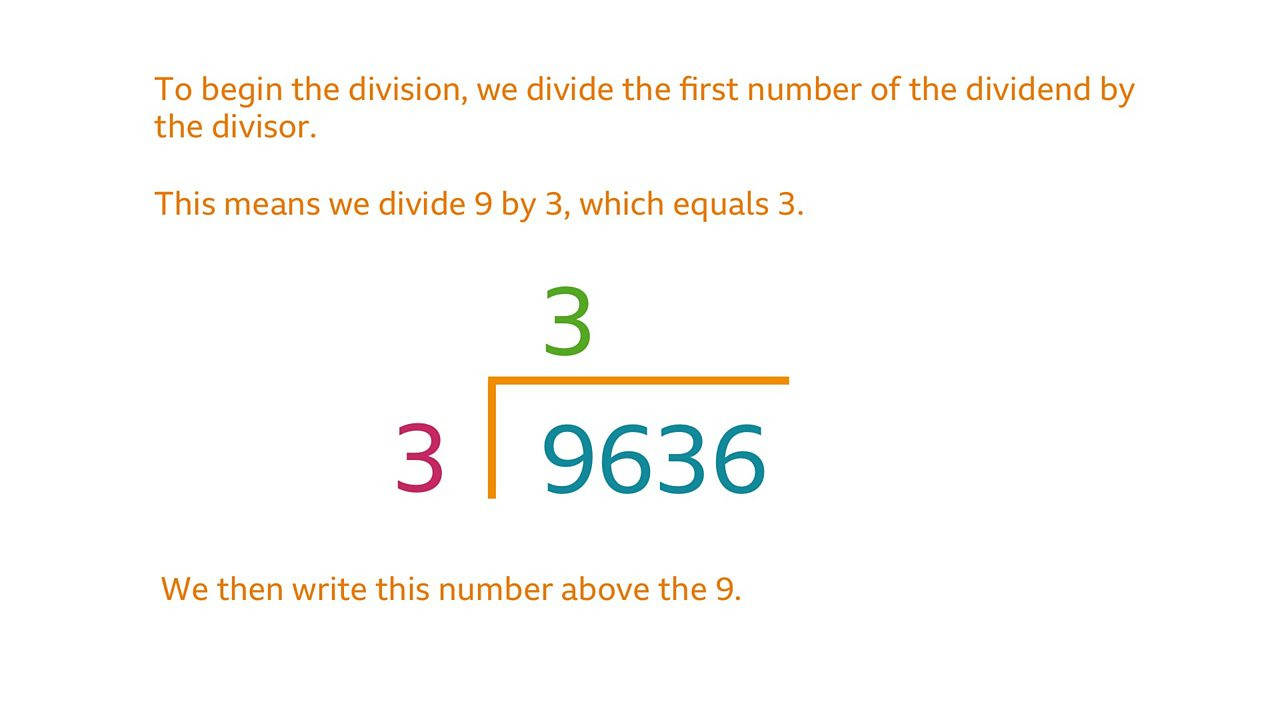To begin with, we divide the first number of the dividend by the divisor, so we divide 9 by 3 which equals = 3. We then write this number above the 9.4 of 8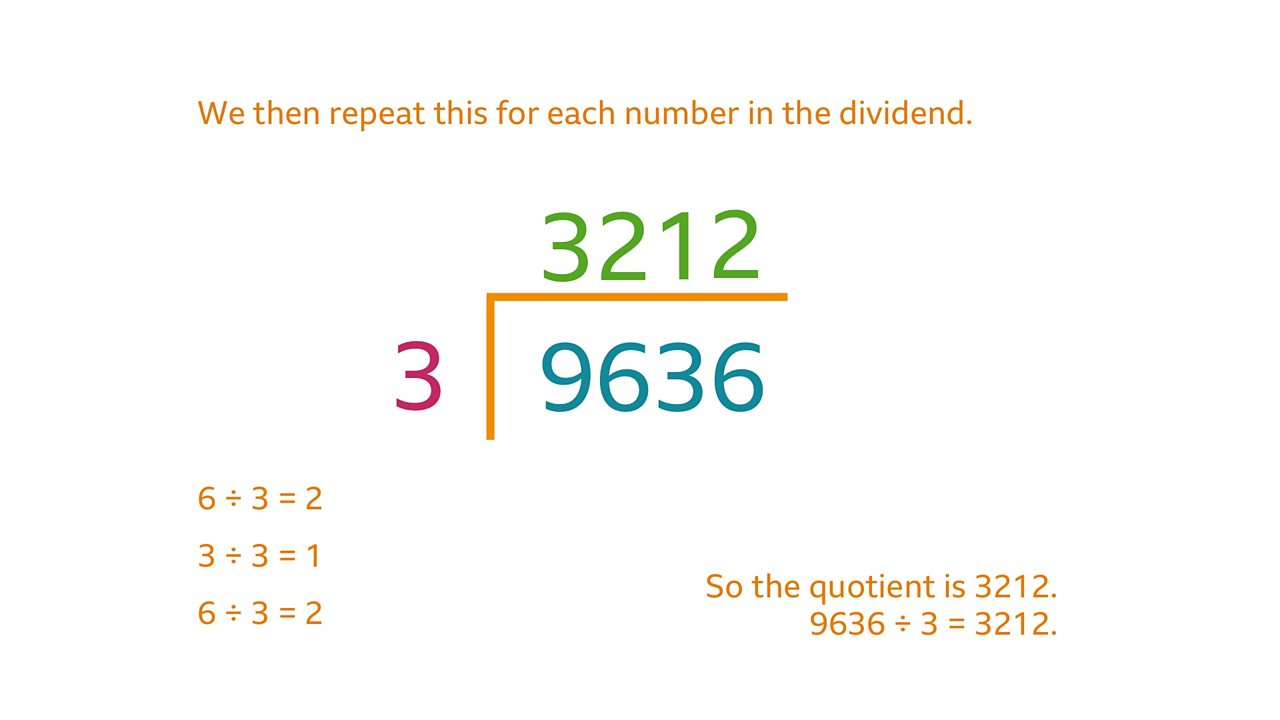We then repeat this for each number in the dividend. 6 ÷ 3 = 2, 3 ÷ 3 = 1, 6 ÷ 3 = 2. So the quotient is 3212. 9636 ÷ 3 = 3212.5 of 8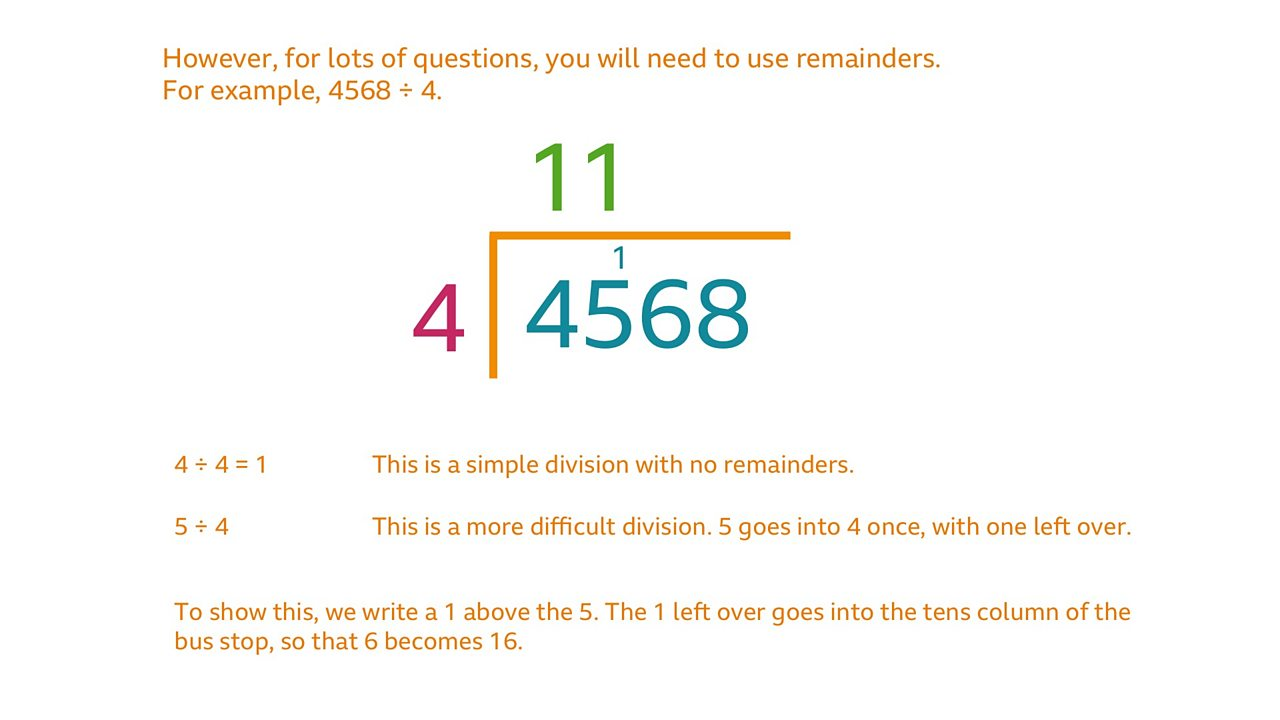For lots of questions, you'll need to use remainders.6 of 8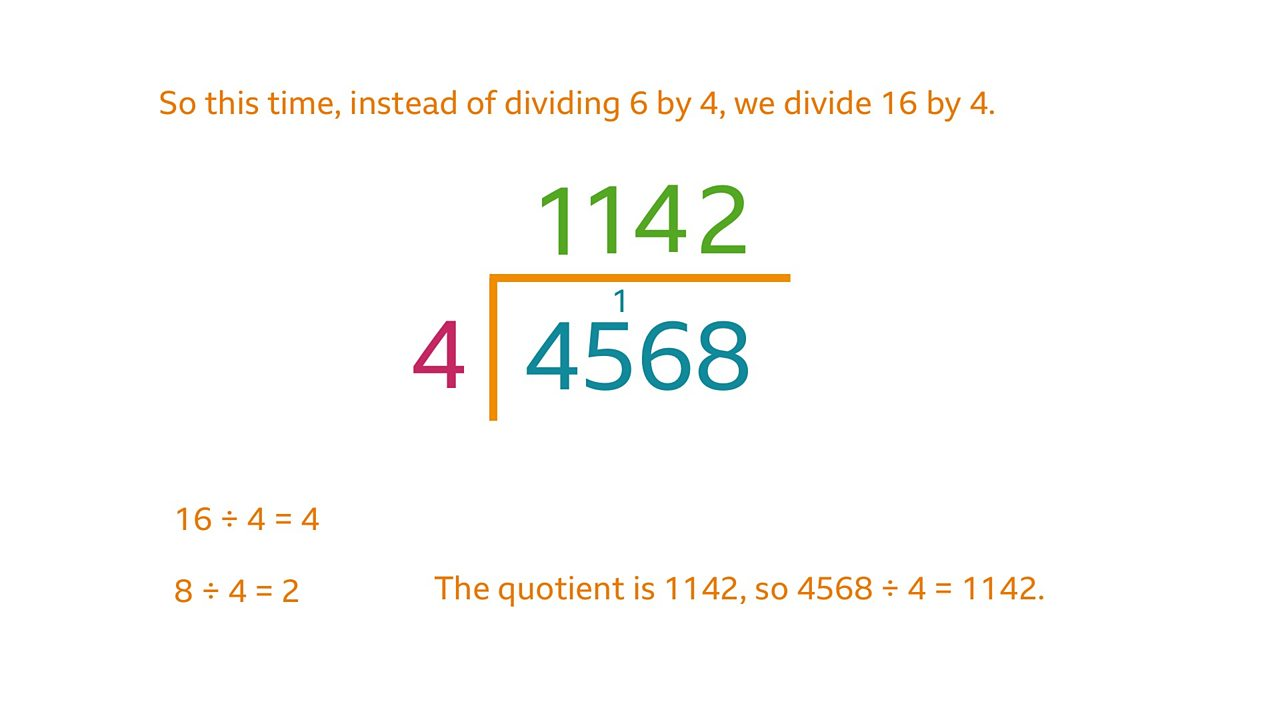7 of 8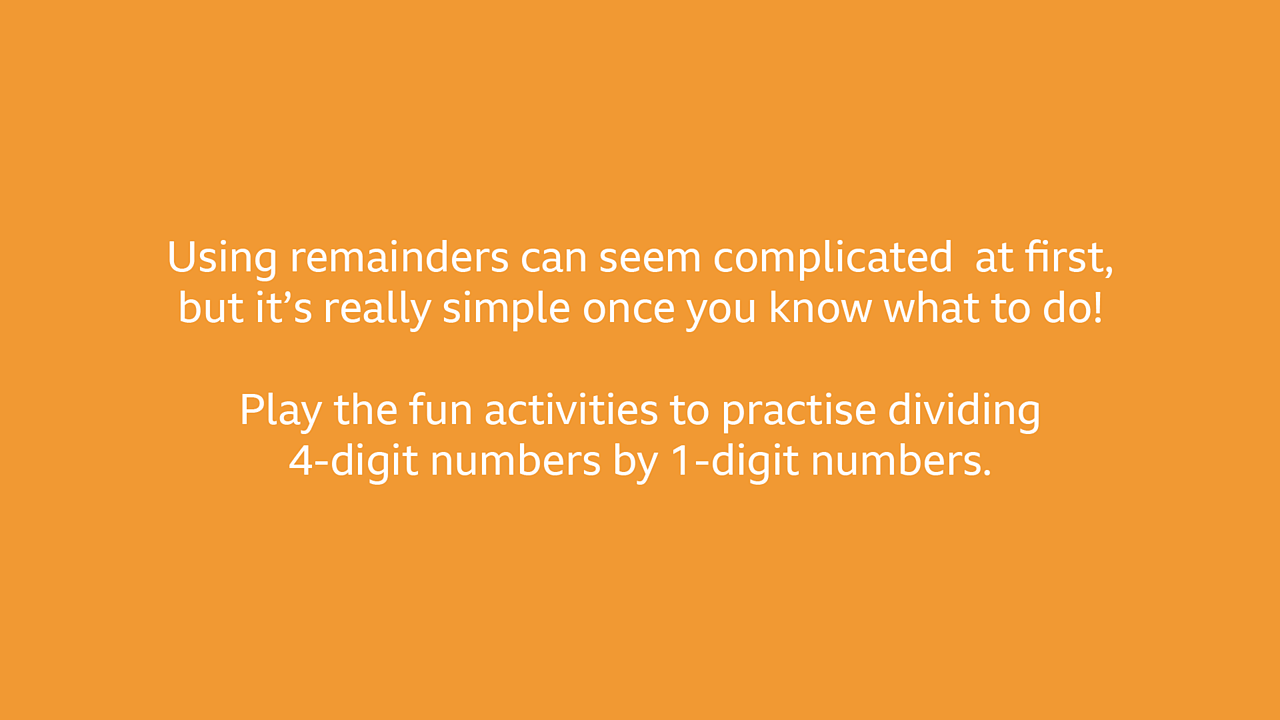Using remainders can seem complicated at first but it's simple once you know what to do. Play the activities to practise.8 of 8

# Practise

## Activity 1

The Greatest Remainder

• The aim of this game is to be the player with the greatest remainder at the end! Take it in turn to roll a dice. Write down your number each time. Roll the dice four times each so that you each have a 4-digit number, such as 2396.

• Now that each of you has a 4-digit number, each player chooses a number between 3 and 9. Divide your 4-digit number by this number.

• The player with the greatest remainder wins, as long as their calculation is correct!

• Extension: Play the game again. This time, the aim is to be the player with the smallest remainder. How will this affect how you play?

## Activity 2

Dice Divisions

• This is a multi-player game. Start your go by rolling a dice. Divide the 4-digit number you are on by the number on the dice. If you manage to divide it correctly, you can move the number of spaces you rolled on the downloadable board below. Then it is your opponent’s turn.

• Take it in turns to move around the track. The first player to reach the end wins!